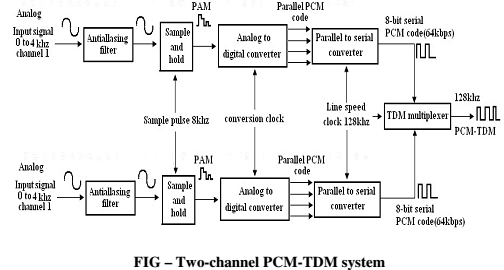## How speech is transmitted in digital switching environment, Electrical Engineering

Assignment Help:

Q. How speech is transmitted in digital switching environment by using PCM/TDM?

Ans:

A digital carrier system is a communications system which uses digital pulses instead of analogue  signals  to  encode  information.  Figureshows  the  block  diagram  for  a digital  carrier  system.  This  digital  carrier  system T1  employs PCM-encoded  samples from  24  voice  band  channels  for  transmission  over  an optical  fibre  transmission  line  or single  metallic  wire  pair.  Every voice-band channel  has  a  band  width  of about 300 Hz to 3000 Hz. Again, multiplexer is simply a digital switch with  24  independent  inputs  and  one  time  division  multiplexed  output.  PCM output  signals  from  24  voice-band  channels  are  sequentially  selected  and connected through multiplexer to transmission line.

When  a  T1  carrier  system,  D-type  (digital)  channel  banks  perform  sampling, multiplexing  and  encoding  of  24  voice-band  channels,   every channel  comprise an eight-bit PCM code and is sampled 8000 times a second. Every channel is sampled at the same rate. Figuredemonstrates the channel sampling sequence for a 24-channel T1 digital carrier system. As the Figureillustrates each channel is sampled once each frame though not at the same instance. Every channel's sample is offset from previous channel's sample by  1/24  of  the  total  frame  time.  Hence  one  64-kbps  PCM-encoded  sample  is transmitted for every voice-band channel during each frame (a frame time of 1/8000 = 125 µs). Afterwards, an extra bit (known as the framing bit) is added to every frame. Framing  bit  occurs  once  per  frame  (8000-bps  rate)  and  is  recovered  in the receiver, where it's used to maintain frame and sample synchronization between TDM receiver andtransmitter.#### DC generators, 3. Why the external characteristics of a DC shunt generator ...

3. Why the external characteristics of a DC shunt generator is more drooping than that of a separately excited generator?

#### Induced emf, applications of dynamically induced emf

applications of dynamically induced emf

#### Determine the voltage in given circuit, Q. In the circuit shown in Figure w...

Q. In the circuit shown in Figure with an ideal op amp, find v o as a function of v a and v b .

#### find the thevenin and norton equivalent circuits, The output port of the o...

The output port of the one-port is defined by terminals A and B. Given: R 1 = 10 k_, R 2 = 20 k_, R 3 = 10 k_, and R 4 = 10 k_ V 1 = 20 V and I1 = 0.8 mA a) Find the T

#### Thermal –root , A moving coil galvanometer consists of a coil  in a uniform...

A moving coil galvanometer consists of a coil  in a uniform magnetic field B o, suspended fro a fibre of torque constant C, current I produces a deflection O=nAB o i/c where n is

#### Sinusoidal inverter, i am going to make a sinusoidal inverter as my final y...

i am going to make a sinusoidal inverter as my final year project kindly help me in this regard.How can i make this project?

#### Explain thevenin''s theorem procedure, Thevenin's theorem procedure:   1. ...

Thevenin's theorem procedure:   1.  Open circuit R L and find Thevenin's voltage (V TH ). 2.  Find Thevenin's resistance (R TH ) when voltage source is short circuit or curren

#### Radio over Fiber, I need to get a summary for a radio over fiber paper that...

I need to get a summary for a radio over fiber paper that I have , the summary need to be on the following format: abstract, introduction,methodology,results, conclusion and refere

#### What are the modes of operations used in 8253, What are the modes of operat...

What are the modes of operations used in 8253? Each of the three counters of 8253 can be operated in one of the following six modes of operation. 1. Mode 0 (Interrupt on ter

#### Measure and set frequency by using an oscilloscope, 1. Introduction : ...

1. Introduction : Theory: Frequency is the number of occurrences of a repeating event per unit time. It can also be defined as the number of repetitions (cycles) of a w